Maxwell's Demon: Flash game with the estimation of the entropy

Yuriy Parfenov

ABSTRACT

The thought experiment "Maxwell's demon" is realized in Flash game. The estimation of the entropy of the system of molecules is performed in the game. The game is developed by free Actionscript compiler FlashDevelop

Maxwell's demon is a thought experiment formulated by James Clerk Maxwell to study the possibility of violating the second law of thermodynamics. According to the second law of thermodynamics the entropy (measure of the order or chaos) of the isolated system is tending to increase over time, approaching a maximum value at equilibrium. The maximum value of the entropy corresponds to the most probable configuration of the system. Let us consider, for example, vessel filled with two different types of molecules. The molecules of the first type are moving faster than molecules of the second type. The most probable configuration (equilibrium) corresponds to the uniform mixture of these molecules. If at the initial moment the fast molecules are located in one part of the vessel and slow molecules are located in the other part, the configuration of the system is changing into the uniform mixture and the entropy of the system will increase. Now let us suppose that such a vessel is divided into two portions by the wall with the door that can be opened by the demon. Demon will let pass fast molecules to one part of the vessel and slow molecules to another. Due to this sorting the distribution of the molecules in the vessel is changing to less probable configuration and the entropy of the system is decreasing. The results of the thought experiment seem to contradict to the second law of thermodynamics. However, more thorough analysis of the whole system including demon shows, that demon must generate more entropy segregating the molecules than it could ever eliminate by the method described.
The thought experiment of J.C.Maxwell was realized in several Flash games. The goal of the game user in these games is to sort out different kinds of the molecules. The decreasing of the entropy of the system of the molecules due to the work of Maxwell's demon is not performed in these games. However, such estimation could be interesting to study this thought experiment more thoroughly.
In the game Maxwell's demon (Screenshot is presented in the Figure 1) the entropy of the system of molecules is estimated at each moment of time by Boltzmann's equation. There are 20 fast molecules (red circles), 20 slow molecules (blue circles) in the vessel. Demon (Smiley in the Figure 1 located in the place of the mouse cursor) can open three doors in the wall in the central part of the vessel by mouse click. If red circles are gathered in one part of the vessel, blue circles in another, the entropy of the system will decrease to 0. The aim of the game is to put entropy of the system of molecules to 0 for the lowest number of mouse clicks. Game is developed by free ActionScript compiler FlashDevelop. Flashdevelop project of the game can be downloaded here:Maxwell's demon project.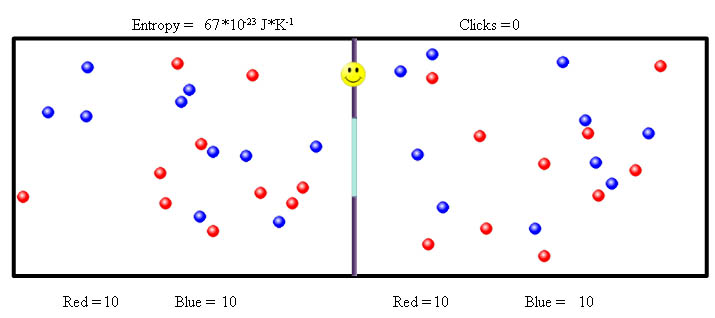Figure 1. Screenshot of the game Maxwell?s demon

Let us consider the vessel which is divided in two equal parts A and B. There are N fast molecules (red balls), N slow molecules (blue molecules). At first, we shall define the entropy for the distribution of the fast molecules. We assume that each part A or B could contain N fast molecules. So we can say that there are N free cells for fast molecules in each part. Let us assume that there are k molecules in the part A. We must define the combination of N identical free cells for k identical molecules. The number of combinations is defined by the binomial coefficient: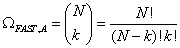As the part A contains k molecules, the part B contains N-k molecules. So, the number of combinations in the part B is defined by the following binomial coefficient: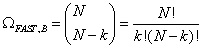Each combination in the part A can be combined to each combination in the part B. So, the total number of combinations for fast molecules (red circles) is the following: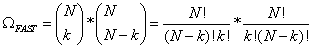We shall assume that the distribution of slow molecules (blue balls) is defined in the same manner and is independent from the distribution of fast molecules. So, the number of combinations of slow molecules is defined as following: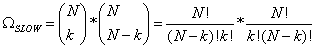The total number is defined as following: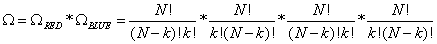In statistical thermodynamics the entropy is defined as (proportional to) the logarithm of the number of microscopic configurations (in our case number of the combinations of molecules) that result in the observed macroscopic description of the thermodynamic system: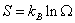where k is Boltzmann's constant.
Hosted by www.Geocities.ws